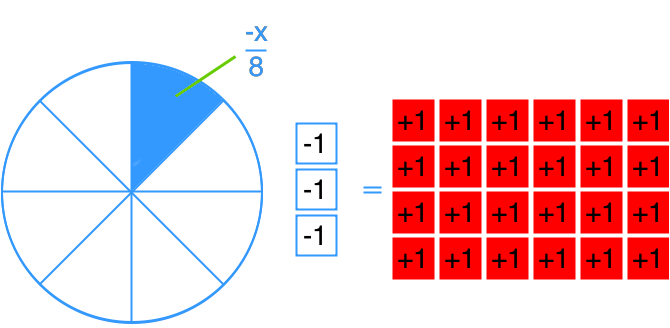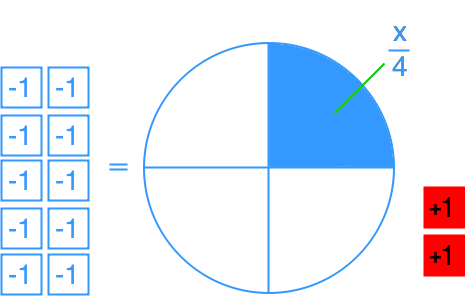# Solving two-step linear equations using multiplication and division: x/a + b = c#### Everything You Need in One Place

Homework problems? Exam preparation? Trying to grasp a concept or just brushing up the basics? Our extensive help & practice library have got you covered.#### Learn and Practice With Ease

Our proven video lessons ease you through problems quickly, and you get tonnes of friendly practice on questions that trip students up on tests and finals.#### Instant and Unlimited Help

Our personalized learning platform enables you to instantly find the exact walkthrough to your specific type of question. Activate unlimited help now!

0/8
##### Examples
###### Lessons
1. Find x in the equation modelled by each diagram.

1.2.2. Solve.
1. $3 + \frac{x}{4} = 51$
2. $17 = 10 + \frac{x}{{ - 2}}$
3. $\frac{x}{{ - 6}} - 21 = - 9$
4. $- 1 = 23 + \frac{x}{8}$
3. The amount of time needed for an ice cube to melt when the temperature is between 10 °C to 30 °C can be expressed by the equation $t = 16 - \frac{C}{2}$, where t is the amount of time for the ice cube to melt in minute; and C is the temperature in degree Celsius.
1. Will the ice melt in 10 minutes if the temperature is 10 °C?
2. What was the lowest temperature for the ice cube to melt in 5 minutes?
0%
##### Practice
###### Topic Notes
Continue from the previous section, we will solve linear equations in diagram, equation, and word problems forms. However, in addition to subtraction and addition, we will teach you how to solve linear equations that also involve multiplications and divisions.